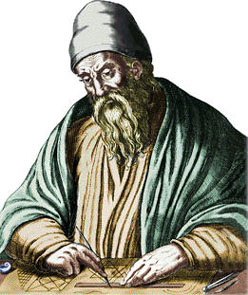Search IntMath
Close

# What did Euclid really say about geometry?

By Murray Bourne, 06 Dec 2010

In school, we all learn about points, lines, 2-D and 3-D shapes, and number theory (prime numbers, etc.)

Sometimes we are told what we are learning is "according to Euclid", but we don't learn very much about where it all comes from.

I recently came across a translation of Euclid's Elements and enjoyed skimming through it (I don't claim to have read all of it - it's over 500 pages!) It is a remarkable collection of knowledge from the 3rd century BCE.

## Euclid's ElementsEuclid constructing a straight line.

You can see the modern translation of Euclid's original here:

Euclid's Elements

(This PDF has a lot of blank pages at the beginning.)

Euclid's Elements is the oldest and most famous mathematical textbook of all time. It forms the basis of much of the mathematics we learn in schools to this day.

The Elements, contrary to popular belief, wasn't original work by Euclid since most of the theorems were by other Greek mathematicians (Aristotle, Eudoxus of Cnidus, Plato, Pythagoras). However, Euclid's contribution was to arrange the axioms, theorems and corollaries in a logical order such that it was easy to reference. He also wrote some of the proofs.

Another misconception is that the Elements is only about geometry. In fact, there is a great deal about number theory as well.

Euclid demonstrated that all the geometrical theorems found in the Elements follow from 5 simple axioms:

Definitions
1. A point is that of which there is no part.
2. And a line is a length without breadth.
3. And the extremities of a line are points.
4. A straight-line is (any) one which lies evenly with points on itself.
5. And a surface is that which has length and breadth only.

Euclid believed you couldn't prove things by measurement - you had to prove them mathematically.

There are 13 Books in the Elements. Some of them are:

1. Fundamentals of Plane Geometry Involving Straight-Lines
2. Fundamentals of Geometric Algebra
3. Fundamentals of Plane Geometry Involving Circles
...
5. Proportion (probably by Eudoxus - includes irrational numbers) (see p135 for proportion diagram)
...
7. Elementary Number Theory (probably from School of Pythagoras)
...
11. Elementary Stereometry (3-D geometry)
...
13. The Platonic Solids
The five regular solids—the cube, tetrahedron (i.e., triangular pyramid), octahedron, icosahedron, and dodecahedron — were problably discovered by the school of Pythagoras. They are generally termed “Platonic” solids because they feature prominently in one of Plato’s dialogues.

## Definitions

The English translation has liberal sprinklings of the following terms and I wanted to make sure I knew the differences between them:

Element - the essential principles
Postulate - a basic principle; a true statement that does not need to be proved
Common Notion - a unit of knowledge
Proposition - a proved and often interesting result, but generally less important than a theorem.
Corollary - a statement that follows with little or no proof required from an already proven theorem.
Lemma - a proved statement that is used as a stepping stone leading on to other more significant results

I hope you enjoy looking through Euclid's Elements as much as I did. It is a remarkable book.

[You may also be interested in What did Newton say about Integration?.]

### Comment Preview

HTML: You can use simple tags like <b>, <a href="...">, etc.

To enter math, you can can either:

1. Use simple calculator-like input in the following format (surround your math in backticks, or qq on tablet or phone):
a^2 = sqrt(b^2 + c^2)
(See more on ASCIIMath syntax); or
2. Use simple LaTeX in the following format. Surround your math with $$ and $$.
$$\int g dx = \sqrt{\frac{a}{b}}$$
(This is standard simple LaTeX.)

NOTE: You can mix both types of math entry in your comment.